# Common Core: 6th Grade Math : Understand Data is Collected to Answer a Question and has a Center, Spread, and Shape: CCSS.Math.Content.6.SP.A.2

## Example Questions

← Previous 1

### Example Question #1 : Understand Data Is Collected To Answer A Question And Has A Center, Spread, And Shape: Ccss.Math.Content.6.Sp.A.2

Select the description that best describes the distribution of the data shown in the provided dot plot.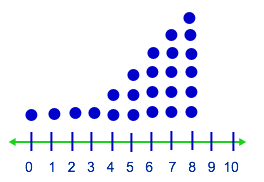Left skewed

Right skewed

Symmetric

Left skewed

Explanation:

In order to answer this question correctly, we need to define our answer options:

Symmetric: A symmetric distribution will have a middle, or center, and each side from the middle will look fairly similar. Many symmetrical distributions are bell shaped, with the middle being tall, and the two sides thinning out.

Left skewed: A left skewed distribution will have most of the data on the right side of the number line.

Right skewed: A right skewed distribution will have most of the data on the left side of the number line.

The data shown in the number plot has most of the data on the right side; thus, left skewed is the correct answer.

### Example Question #2 : Understand Data Is Collected To Answer A Question And Has A Center, Spread, And Shape: Ccss.Math.Content.6.Sp.A.2

Select the description that best describes the distribution of the data shown in the provided dot plot.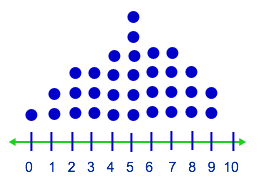Right skewed

Left skewed

Symmetric

Symmetric

Explanation:

In order to answer this question correctly, we need to define our answer options:

Symmetric: A symmetric distribution will have a middle, or center, and each side from the middle will look fairly similar. Many symmetrical distributions are bell shaped, with the middle being tall, and the two sides thinning out.

Left skewed: A left skewed distribution will have most of the data on the right side of the number line.

Right skewed: A right skewed distribution will have most of the data on the left side of the number line.

The data shown in the number plot provided has a bell shape look; thus, symmetric is the correct answer.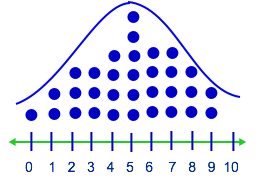### Example Question #1 : Understand Data Is Collected To Answer A Question And Has A Center, Spread, And Shape: Ccss.Math.Content.6.Sp.A.2

Select the description that best describes the distribution of the data shown in the provided dot plot.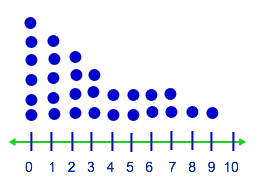Symmetric

Right skewed

Left skewed

Right skewed

Explanation:

In order to answer this question correctly, we need to define our answer options:

Symmetric: A symmetric distribution will have a middle, or center, and each side from the middle will look fairly similar. Many symmetrical distributions are bell shaped, with the middle being tall, and the two sides thinning out.

Left skewed: A left skewed distribution will have most of the data on the right side of the number line.

Right skewed: A right skewed distribution will have most of the data on the left side of the number line.

The data shown in the number plot is mostly on the left side; thus, right skewed is the correct answer.

### Example Question #1 : Understand Data Is Collected To Answer A Question And Has A Center, Spread, And Shape: Ccss.Math.Content.6.Sp.A.2

Select the description that best describes the distribution of the data shown in the provided dot plot.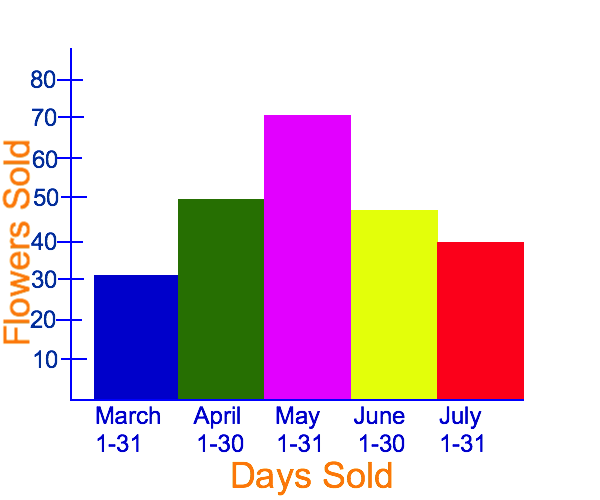Right skewed

Symmetric

Left skewed

Symmetric

Explanation:

In order to answer this question correctly, we need to define our answer options:

Symmetric: A symmetric distribution will have a middle, or center, and each side from the middle will look fairly similar. Many symmetrical distributions are bell shaped, with the middle being tall, and the two sides thinning out.

Left skewed: A left skewed distribution will have most of the data on the right side of the number line.

Right skewed: A right skewed distribution will have most of the data on the left side of the number line.

The data shown in the number plot provided has a bell shape look; thus, symmetric is the correct answer.

### Example Question #1 : Understand Data Is Collected To Answer A Question And Has A Center, Spread, And Shape: Ccss.Math.Content.6.Sp.A.2

Select the description that best describes the distribution of the data shown in the provided dot plot.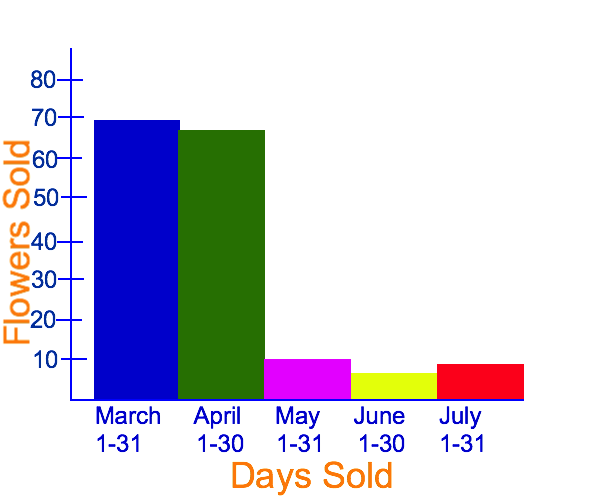Right skewed

Symmetric

Left skewed

Right skewed

Explanation:

In order to answer this question correctly, we need to define our answer options:

Symmetric: A symmetric distribution will have a middle, or center, and each side from the middle will look fairly similar. Many symmetrical distributions are bell shaped, with the middle being tall, and the two sides thinning out.

Left skewed: A left skewed distribution will have most of the data on the right side of the number line.

Right skewed: A right skewed distribution will have most of the data on the left side of the number line.

The data shown in the number plot is mostly on the left side; thus, right skewed is the correct answer.

### Example Question #7 : Understand Data Is Collected To Answer A Question And Has A Center, Spread, And Shape: Ccss.Math.Content.6.Sp.A.2

Mrs. Frame's class counted the number pencils that each student had in his/her desk. The distribution of this data is show in the dot plot provided. What is the center of this distribution?Explanation:

The data in the distribution shows the number of pencils each student found in his/her desk. Each student found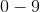pencils in their desks. Based on this, and by looking at the graph, we can see that the enter of the distribution is### Example Question #1 : Understand Data Is Collected To Answer A Question And Has A Center, Spread, And Shape: Ccss.Math.Content.6.Sp.A.2

Mrs. Frame's class counted the number pencils that each student had in his/her desk. The distribution of this data is show in the dot plot provided. What is the most number of pencils that her students found?Explanation:

The dot plot is on a number line that is numbered from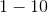is the highest number on the number line, but there are no dots above that number, which means no one foundpencils in their desk.is the next highest number, and there are two dots over the number on the number line; thus, the correct answer is### Example Question #11 : Statistics & Probability

Mrs. Frame's class counted the number pencils that each student had in his/her desk. The distribution of this data is show in the dot plot provided. How many pencils did most of the students have?Explanation:

If we look at the dot plot provided, the most number of dots is above the numberon the number line. There aredots above the number, which means thatstudents foundpencils in their desks, which is the most students for the same number of pencils; thus,is the correct answer.

### Example Question #10 : Understand Data Is Collected To Answer A Question And Has A Center, Spread, And Shape: Ccss.Math.Content.6.Sp.A.2

A flower shop counted the number of flowers sold during amonth period. The distribution of this data is show in the histogram provided. What is the center of this distribution?June

March

April

July

May

May

Explanation:

The data in the distribution shows the number of flowers sold in each month. By looking at the graph, we can see that there arebars representingmonths, and the month of May is in the middles; thus, May is the center of this distribution.

### Example Question #11 : Understand Data Is Collected To Answer A Question And Has A Center, Spread, And Shape: Ccss.Math.Content.6.Sp.A.2

A flower shop counted the number of flowers sold during amonth period. The distribution of this data is show in the histogram provided. What is the most number of flowers the shop sold in a given month?Explanation:

To answer this question, we can look at our graph and see how far each bar in the histogram raises: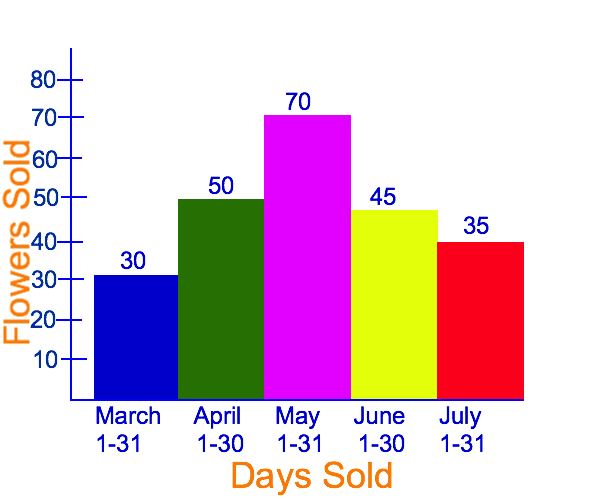We can see that in May, the flower shop soldflowers, which is the most flowers that were sold in a given month.

← Previous 1

### All Common Core: 6th Grade Math Resources